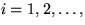Next: Line graphs - vector Up: IBIS postprocessing file formats: Previous: Line graphs - fluid

### Line graphs - scalar variables:

Line Graphics Scalar File Format.

This file contains the values of the computed scalar fields. The header file defines the number of data points in this file.

The file must contain nxyfs rows and nfs columns. The precise format of each piece of data is not critical, but the ordering of the data is critical. The file format is as follows.

```  s1(i*h,j*h)  s2(i*h,j*h)  ...
s1(i*h+h,j*h)  s2(i*h+h,j*h)  ...
...
```

Notes:

1.
Each column corresponds to a scalar field. The first number in each row is the first scalar field, the second number is the second scalar field and so on.

2.
Each rows corresponds to a point in the domain where the scalar fields are computed. The data are listed row-wise (left to right) from the bottom to the top. Therefore, the first nx rows are the scalars along the bottom row (y=ymin and x=i*h, wherenx). The next nx scalars correspond to the second row (y=ymin+h and x=i*h, where wherenx), and so on. The last nx vectors correspond to the final row.Next: Line graphs - vector Up: IBIS postprocessing file formats: Previous: Line graphs - fluid
David Eyre
6/19/1998